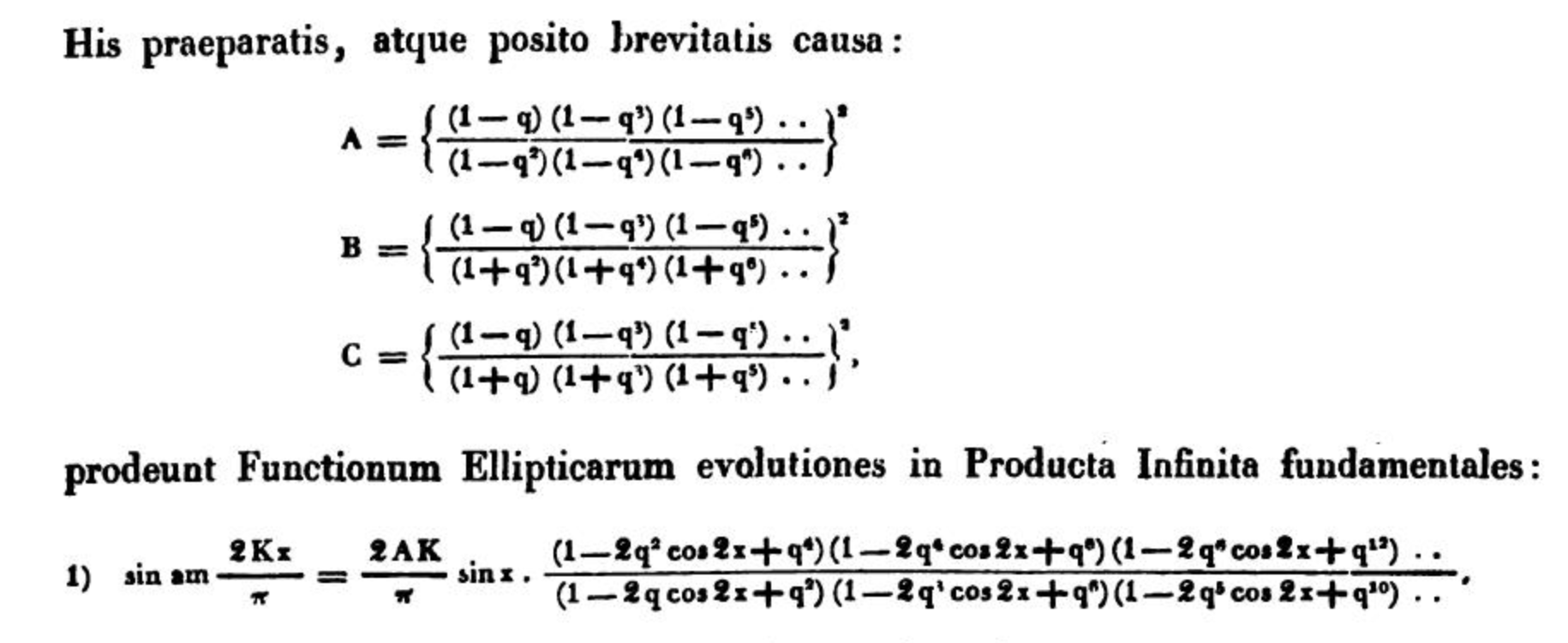# q-difference equations and quantum mechanics

I have been trying to understand why the term quantum is so easily accepted for calculus based on q-numbers $$[n]_q=\frac{q^n-1}{q-1}$$ and q-analogs of classical operators (derivatives, integrals,...).

But the best hints I could find is this question Why are quantum groups so called? this one Intuition behind the definition of quantum groups, and this answer of Pavel Etingof on mathoverflow What is the relation between quantum symmetry and quantum groups?.

But I could not find any attempt to connect the q-calculations arising from the mathematical idea of deformation (in algebra or combinatorics) to precise concepts in quantum mechanics. Have there ever been actual uses of q-calculus and quantum groups to computing or understanding solutions of Schrodinger equations, or functions actually arising from (physical) quantum mechanics, like states of the harmonic oscillator or some simple atomic hamiltonian like that of the hydrogen atom?

Pavel Etingof says that "the main mechanisms through which quantum groups appear in physics is the same as for usual Lie groups" but I have seen hundreds of physics books with applications of classical Lie groups (classical groups usually, $$SO,U,SU,...$$) to quantum mechanical problems but none for quantum groups.

Also when did the term q-calculus, quantum calculus, q-hypergeometric series first appear -in particular in what order did they appear?

Thank you.

• Sometimes the q is not measuring quantum behavior of some physical system. Consider the example of using Bethe ansatz on the XXZ spin chain. The q there measures difference from the isotropic case, not related to $h$. Elsewhere $q=e^h$ is a more illucidating choice of notation. May 22, 2019 at 16:16
• Indeed, for quantum groups the terminology is related to the philosophy that quantisation turns commutative structures into non-commutative ones. Here (the universal enveloping algebra of the Lie algebra for) ordinary symmetries are deformed to be non co-commutative --- or, dually, the algebra of functions on it is made non-commutative. But this doesn't mean that the undeformed object describes classical physics, cf the above example of the XXZ (q=1: XXX) spin chain. It turns out that q-numbers naturally appear (eg in Serre relations), which is perhaps why they are sometimes called "quantum". May 23, 2019 at 1:35
• Sep 3, 2019 at 13:29

There exist applications of q-calculus to physics, but there is no direct relation to quantum mechanics. You can find an overview of some of these applications in q-Calculus and physics (paywall).

This should not be a surprise, because the "q" in q-calculus does not stand for "quantum", at least it did not originally. It predates quantum mechanics by two centuries and it is believed that the "q" was originally used (by Euler?) as an abbreviation of "quotient". The history is described by T. Ernst in The different languages of q-calculus.

Sources: The cited article by Ernst says (on page 39): "Euler had in fact already introduced q-series and Jacobi continued to use the letter q, which has survived until today."
As far as I could check, Euler used $$x$$ instead of $$q$$ (for example, in Evolutio producti infiniti), but Jacobi did indeed use $$q$$. Here is Jacobi's q-series from Fundamenta nova theoriae functionum ellipticarum (1829, page 86):The systematic theory of q-series started with E. Heine (1847).

• Thanks, in that book I could find a reference to iopscience.iop.org/article/10.1088/0954-3899/16/8/006/pdf which finds a mildly physical application to "deformed nuclei". But it is definitely not much.
– plm
May 22, 2019 at 16:13
• Also, do you have a reference for Euler's use of the term? I saw that he used the concept, I am trying to see who introduced the actual term and envisioned a theory for it.
– plm
May 22, 2019 at 16:15

Regarding the first part of the question:

Have there ever been actual uses of q-calculus and quantum groups to computing or understanding solutions of Schrodinger equations, or functions actually arising from (physical) quantum mechanics, like states of the harmonic oscillator or some simple atomic hamiltonian like that of the hydrogen atom?

In my understanding, the answer is yes, there have been lots of applications in various different settings coming from quantum mechanical problems. As far as i know, the first applications of this kind were the algebraic descriptions of the solutions of the Schrodinger equations for (deformed) $$q$$-oscillators and $$q$$-rotators together with the computations of the corresponding energy spectrums, transition rates etc. Among the pioneering papers:

Since then, there has been quite a lot of literature on similar topics. If we confine ourselves to low and intermediate energy QM (that is: mainly models of atomic and nuclear physics), an early overview can be found at:

For a more complete list and further discussion on "actual uses of $$q$$-calculus and quantum groups ... ", maybe you will be interested in the answers (and the references included) to the following question: